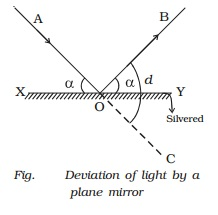Home | | Physics | Reflection of light

# Reflection of lightHighly polished metal surfaces reflect about 80% to 90% of the light incident on them. Mirrors in everyday use are therefore usually made of depositing silver on the backside of the glass.

Reflection of light

Highly polished metal surfaces reflect about 80% to 90% of the light incident on them. Mirrors in everyday use are therefore usually made of depositing silver on the backside of the glass. The largest reflector in the world is a curved mirror nearly 5 metres across, whose front surface is coated with aluminium. It is the hale Telescope on the top of Mount Palomar, California, U.S.A. Glass by itself, will also reflect light, but the percentage is small when compared with the case of silvered surface. It is about 5% for an air-glass surface.

1.Laws of reflection

Consider a ray of light, AO, incident on a plane mirror XY at O. It is reflected along OB. Let the normal ON is drawn at the point of incidence. The angle AON between the incident ray and the normal is called angle of incidence, i (Fig.) the angle BON between the reflected ray and the normal is called angle of reflection, r. Experiments show that :

(i) The incident ray, the reflected ray and the normal drawn to the reflecting surface at the point of incidence, all lie in the same plane.

(ii) The angle of incidence is equal to the angle of reflection. (i.e) i = r.

These are called the laws of reflection.2.Deviation of light by plane mirror

Consider a ray of light, AO, incident on a plane mirror XY (Fig.) at O. It is reflected along OB. The angle AOX made by AO with XY is known as the glancing angle α with the mirror. Since the angle of reflection is equal to the angle of incidence, the glancing angle

BOY made by the reflected ray OB with the mirror is also equal to α.

The light has been deviated from a direction AO to a direction OB. Since angle COY = angle AOX, it follows that angle of deviation, d = 2α

So, in general, the angle of deviation of a ray by a plane mirror or a plane surface is twice the glancing angle.3.Deviation of light due to rotation of a mirror

Let us consider a ray of light AO incident on a plane mirror XY at O. It is reflected along OB. Let α be the glancing angle with XY (Fig.). We know that the angle of deviation COB = 2α.

Suppose the mirror is rotated through an angle θ to a position X′Y′.

The same incident ray AO is now reflected along OP. Here the glancing angle with X′Y′ is (α + θ). Hence the new angle of deviation COP = 2 (α + θ). The reflected ray has thus been rotated through an angle BOP when the mirror is rotated through an angle θ.Angle BOP  = Angle COP  = Angle COB

Angle BOP = 2 (α + θ) ? 2α = 2θ

For the same incident ray, when the mirror is rotated through an angle, the reflected ray is rotated through twice the angle.

Study Material, Lecturing Notes, Assignment, Reference, Wiki description explanation, brief detail
11th 12th std standard Class Physics sciense Higher secondary school College Notes : Reflection of light |